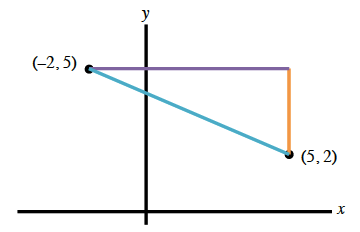### Home > A2C > Chapter 1 > Lesson 1.2.1 > Problem1-62

1-62.

Consider the points $(−2,5)$ and $(5,2)$ as you complete parts (a) and (b) below

1. Plot the points and find the distance between them. Give your answer both in simplest radical form and as a decimal approximation.

Refer to problem 1-49.2. Find the slope of the line that goes through the two points.

Plot the points on the graph using the eTool below. Find the distance and slope.
Click the link at right for the full version of the eTool:  1-62 HW eTool